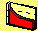3.1 OVERVIEW

Chapter 2, Portfolio Statistics, shows you how to quantify both risk and return of a portfolio. The expected return is measured as the mean or average return of the portfolio. The risk is measured by the standard deviation of portfolio returns. In this chapter, we study the effects of increasing the number of stocks on the risk of a portfolio.

Suppose you have money to invest in the stock market. Intuitively, you may realize that investing in many stocks is likely to reduce the variability of returns from your portfolio compared to, say, investing in only a single stock. The act of reducing risk by investing in many stocks is called diversification.

But how much diversification is desirable? In practice, the more securities you acquire, the higher your transaction costs become, but in our theoretical explanation, we assume away frictions such as transaction costs. This lets us measure the effects of diversification, and assess the desirability of diversification, in a perfect market.

In fact, measuring the benefits from diversification is a major component of modern portfolio theory. In this chapter, we measure the benefits from diversification using a simple approach called naive diversification. This approach allocates equal amounts of wealth to each security in your portfolio.

We analyze the effects of naive diversification from both a statistical/analytical perspective and an empirical perspective. The approach gives us additional insight into the relative nature of risk. You will find that a security contributes to portfolio variance in two ways : through its variance and its covariance with other securities. As you increase the number of securities, the variance part can be reduced or eliminated. Covariance terms, however, remain even when the portfolio gets very large. The contribution to portfolio risk due to the variance terms alone is sometimes called diversifiable risk. The capital asset pricing model classifies risk into systematic and unsystematic risk, as you will see in other chapters.

In topic 3.2, Equally Weighted Portfolios, we review empirical evidence about the effect on portfolio risk of increasing the number of stocks. The question is: do we need to form portfolios of 10,000 securities, or 100 or fewer securities, to obtain the benefits from naive diversification? As you will see, many of the benefits can be obtained by holding as few as 15 to 20 securities. We also analyze naive diversification formally. We explain the different ways a security contributes to portfolio risk, which lead you to think about portfolios other than equally weighted ones.

In an example, we apply the concept of naive diversification to the Three-Firm Case. CAPM Tutor lets you see for yourself how naive diversification reduces portfolio variance, whether you use the default Three-Firm Case data set or other data sets with many more firms.

previous topic

next topic